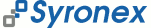Home | Search | Contact

# Advanced Oracle & Java

Binary large objects — Java stored procedures — call specifications — triggers.

#### Introduction

You have been reviewed so far JDBC basics. In the next sections, we will discuss two more advanced topics: how to run PL/SQL code from Java—and conversely, how to run Java code from PL/SQL. The two issues we are going to address during this laboratory have a larger scope than the ones in the previous laboratories.

#### Java versus PL/SQL.

The considerations listed below outline why and when to use Java rather than PL/SQL:
• Java offers more opportunities for reuse accross applications (c.f. class Mod11Ck below)
• there are more Java programmers that PL/SQL programmers
• Java is potentially more efficient for non-database related programmative tasks
• PL/SQL is a proprietary language only supported by Oracle.
By constrast, PL/SQL has the following advantages:
• PL/SQL is tightly integrated with the Oracle DBMS, therefore (argueably) easier to use for database applications
• it is more efficient for database-oriented programmative tasks.

## BLOBs & Java

#### Binary Large Objects (BLOBs).

BLOBs are non-atomic, unstructured blocks of binary data stored within a database. We will see in this section how BLOBs can be used to store pictures—and in the next section how Oracle uses BLOBs to store Java classes. As shown below, there exists a BLOB data type in Oracle.
CREATE TABLE pic_store
(
description VARCHAR2(50),
picture BLOB
)
/

Because of the nature of BLOBs, they own a specific INSERT procedure: an empty BLOB is first created using the Oracle built-in function empty_blob(). A stream is then established to the Oracle server to upload the data.

#### Putting it to work...

The two classes in PicDispClasses.jar display pictures stored as BLOBs in Oracle. To avoid overloading the database, you will all use the same table pic_store and account scott/tiger1. Extract the source and class files from the jar archive and execute the program as follows:
jar xvf PicDispClasses.jar

java PicDisp <picture_description>

replacing <picture_description> by one of the values of the description field in the pic_store table2. Once the code is running, make sure you read and understand at least the database-related parts of the source code in PicLoader.java.

## Java Stored Procedures

We will now write a stored procedure that validates ISBN numbers using the modulo 11 check-digit algorithm (explained in appendix). A trigger (called rules in Postgres) will then be created to apply the check whenever data is inserted to the books table (c.f. active database concept). The validation procedure we will use only involves numerical computation, but no data-based processing: it is therefore a good candidate to be written in Java. Moreover, the java class once implemented can be reused in other database and non-database related applications. The Mod11Ck Java class below calculates the check-digit.
public class Mod11Ck {
public static String calc(String digStr) {
int len = digStr.length();
int sum = 0, rem = 0;
int[] digArr = new int[len];
for (int k=1; k<=len; k++) // compute weighted sum
sum += (11 - k) * Character.getNumericValue(digStr.charAt(k - 1));
if ((rem = sum % 11) == 0) return "0";
else if (rem == 1) return "X";
else return (new Integer(11 - rem)).toString();
}
}


Compile the Java class above and load it into Oracle by issuing the following command:

comp-load-j ./Mod11Ck.java


Note that this is not a standard Oracle utility, but a script that I wrote to read your Oracle username and password from the DbObject.conf file (see Introduction to Oracle & Java.) Be aware that DbObject.conf is expected to be in the current directory.
You should be able to lookup the data dictionary to find out whether the Java class compiled successfully (see Oracles's Data Dictionary.) The class will be stored in compiled format as a BLOB, in the database.
Next, we need to write a call specification to publish our java (static) method as a PL/SQL function.

CREATE OR REPLACE FUNCTION check_isbn (isbn VARCHAR2) RETURN VARCHAR2
AS LANGUAGE JAVA
NAME 'Mod11Ck.calc(java.lang.String) RETURN java.lang.String';
/


We can now write a trigger (c.f. rules in Postgres) that will validate the ISBN for each INSERT statement executed on the books table. If the last digit of the ISBN about to be inserted does not match the calculated check-digit, an exception is raised—leading the INSERT statement to be rolled-back.

CREATE OR REPLACE TRIGGER check_isbn_on_ins
BEFORE INSERT ON books
FOR EACH ROW
DECLARE
new_isbn VARCHAR2(10);
BEGIN
new_isbn := TO_CHAR(:NEW.isbn);
IF NOT (LENGTH(new_isbn) = 10 AND
SUBSTR(new_isbn,10,1) = check_isbn(SUBSTR(new_isbn,1,9)))
THEN
RAISE_APPLICATION_ERROR(-20000, 'The ISBN number supplied is invalid!');
END IF;
END;
/


Try to understand how the trigger works.

## Appendix: The Modulo 11 Check-Digit Algorithm

ISBNs are often handled manually and there is therefore a need for a quick way to check whether a particular ISBN is likely to be valid or not. A typical ISBN is represented below, starting with a country code (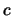), followed by a publisher code () and a title code (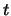). The tenth digit (; called check-digit) depends on all the others. So if there is an alteration in one or more of the first nine digits—and the check-digit is re-calculated, its value is very likely to be different from its original value.The modulo-11 check digit algorithm given below is implemented in the Java class Mod11Ck.

1. [Compute weighted sum.]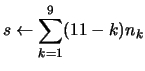where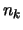is the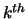digit in the ISBN.
2. [Get remainder.] Divide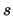by 11 and let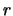be the remainder.
3. [Find check-digit.]

.1.
[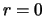?] If, set. The algorithm terminates
.2.
[?] If, set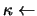`X'. The algorithm terminates.
.3.
[Else.] If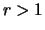, set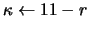. The algorithm terminates.

#### Footnotes

...scott/tiger1
So don't forget to login as scott/tiger.
... table2
Note that you cannot display the content of a column of type BLOB in SQL*Plus.